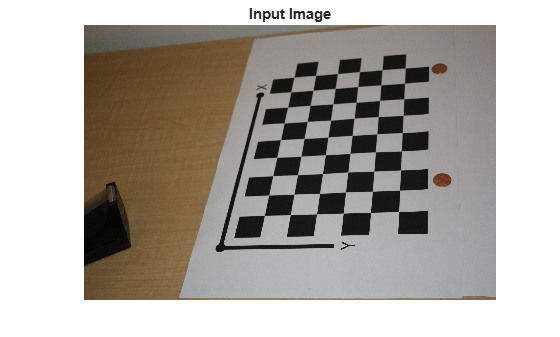# cameraMatrix

(Not recommended) Camera projection matrix

`cameraMatrix` is not recommended. Use the `cameraProjection` function instead. For more information, see Compatibility Considerations.

## Syntax

``camMatrix = cameraMatrix(cameraParams,tform)``
``camMatrix = cameraMatrix(cameraParams,rotationMatrix,translationVector)``

## Description

````camMatrix = cameraMatrix(cameraParams,tform)` returns a 4-by-3 camera projection matrix `camMatrix`, which can be used to project a 3-D world point in homogeneous coordinates into an image. `cameraParams` can be a `cameraParameters` object or a `cameraIntrinsics` object.```

example

````camMatrix = cameraMatrix(cameraParams,rotationMatrix,translationVector)` returns a 4-by-3 camera projection matrix. You can use this matrix to project 3-D world points in homogeneous coordinates into an image.```

## Examples

collapse all

Create a set of calibration images.

```images = imageDatastore(fullfile(toolboxdir('vision'),'visiondata', ... 'calibration','slr'));```

Detect the checkerboard corners in the images.

`[imagePoints,boardSize] = detectCheckerboardPoints(images.Files);`

Generate the world coordinates of the checkerboard corners in the pattern-centric coordinate system, with the upper-left corner at (0,0). The square size is in millimeters.

```squareSize = 29; worldPoints = generateCheckerboardPoints(boardSize,squareSize);```

Calibrate the camera.

```I = readimage(images,1); imageSize = [size(I,1),size(I,2)]; cameraParams = estimateCameraParameters(imagePoints,worldPoints, ... 'ImageSize',imageSize);```

```imOrig = imread(fullfile(matlabroot,'toolbox','vision','visiondata', ... 'calibration','slr','image9.jpg')); figure; imshow(imOrig); title('Input Image');```Undistort image.

`im = undistortImage(imOrig,cameraParams);`

Find reference object in new image.

`[imagePoints,boardSize] = detectCheckerboardPoints(im);`

Compute new extrinsics.

```[rotationMatrix,translationVector] = extrinsics(... imagePoints,worldPoints,cameraParams);```

Calculate camera matrix

`P = cameraMatrix(cameraParams,rotationMatrix,translationVector)`
```P = 4×3 105 × 0.0157 -0.0271 0.0000 0.0404 -0.0046 -0.0000 0.0199 0.0387 0.0000 8.9399 9.4399 0.0072 ```

## Input Arguments

collapse all

Camera parameters, specified as a `cameraParameters` or `cameraIntrinsics` object. You can return the `cameraParameters` object using the `estimateCameraParameters` function. The `cameraParameters` object contains the intrinsic, extrinsic, and lens distortion parameters of a camera.

Transformation from world coordinates to camera coordinates, specified as a `rigid3d` object. You can use the `extrinsics` function to obtain the rotation and translation to create the `tform` object.

Rotation of camera, specified as a 3-by-3 matrix. You can obtain this matrix using the `extrinsics` function. You can also obtain the matrix using the `relativeCameraPose` function by transposing its `orientation` output. The `rotationMatrix` and `translationVector` inputs must be real, nonsparse, and of the same class.

Translation of camera, specified as a 1-by-3 vector. The translation vector describes the transformation from the world coordinates to the camera coordinates. You can obtain this vector using the `extrinsics` function. You can also obtain the vector using the `location` and `orientation` outputs of the `relativeCameraPose` function:

• `translationVector` = -`relativeLocation` * `relativeOrientation'`

The `translationVector` inputs must be real, nonsparse, and of the same class.

## Output Arguments

collapse all

Camera projection matrix, returned as a 4-by-3 matrix. The matrix contains the 3-D world points in homogenous coordinates that are projected into the image. When you set `rotationMatrix` and `translationVector` to `double`, the function returns `camMatrix` as `double`. Otherwise it returns `camMatrix` as `single`.

The function computes `camMatrix` as follows:

`camMatrix` = [`rotationMatrix`; `translationVector`] × K.
K: the intrinsic matrix

Then, using the camera matrix and homogeneous coordinates, you can project a world point onto the image.

w × [x,y,1] = [X,Y,Z,1] × `camMatrix`.

 (X,Y,Z): world coordinates of a point (x,y): coordinates of the corresponding image point w: arbitrary scale factor

Data Types: `single` | `double`

## Version History

Introduced in R2014b

collapse all

### R2022b: Not recommended

Starting in R2022b, most Computer Vision Toolbox™ functions create and perform geometric transformations using the premultiply convention. However, the `cameraMatrix` function uses the postmultiply convention. Although there are no plans to remove `cameraMatrix` at this time, you can streamline your geometric transformation workflows by switching to the `cameraProjection` function, which supports the premultiply convention. For more information, see Migrate Geometric Transformations to Premultiply Convention.

• Change instances of the function name `cameraMatrix` to `cameraProjection`.

• Specify the `cameraParams` argument as a `cameraIntrinsics` object. If you have a `cameraParameters` object, then you can get a `cameraIntrinsics` object by querying the `Intrinsics` property. If the `Intrinsics` property is empty according to the `isempty` function, then set the `ImageSize` property of the `cameraParameters` object to an arbitrary vector before querying the `Intrinsics` property. For example:

```load worldToImageCorrespondences.mat if(isempty(cameraParams.Intrinsics)) cameraParams.ImageSize = [128 128]; end intrinsics = cameraParams.Intrinsics;```
• Specify the transformation as a `rigidtform3d` object using the `tform` argument. `cameraProjection` does not support the `rotationMatrix` and `translationVector` input arguments. Note that you create the `rigidtform3d` object using the transpose of `rotationMatrix` or the transpose of the transformation matrix in the `T` property of `tform`.

Discouraged UsageRecommended Replacement

This example specifies the transformation as a rotation matrix and a translation vector, then calculates the camera projection matrix using the `cameraMatrix` function.

`camMatrix = cameraMatrix(cameraParams,rotationMatrix,translationVector)`

This example specifies the transformation as a `rigidtform3d` object, then calculates the camera projection matrix using the `cameraProjection` function. Note that you create the `rigidtform3d` object using the transpose of the rotation matrix, `rotationMatrix`.

```intrinsics = cameraParams.Intrinsics; tform = rigidtform3d(rotationMatrix',translationVector); camMatrix = cameraProjection(intrinsics,tform);```

This example specifies the transformation as a `rigid3d` object.

`camMatrix = cameraMatrix(cameraParams,tformOld);`

This example starts with an existing `rigid3d` object `tformOld`, creates a `rigidtform3d` object using the transpose of the `T` property of `tformOld`, then calculates the camera projection matrix using the `cameraProjection` function.

```intrinsics = cameraParams.Intrinsics; tform = rigidtform3d(tformOld.T'); camMatrix = cameraProjection(intrinsics,tform);```